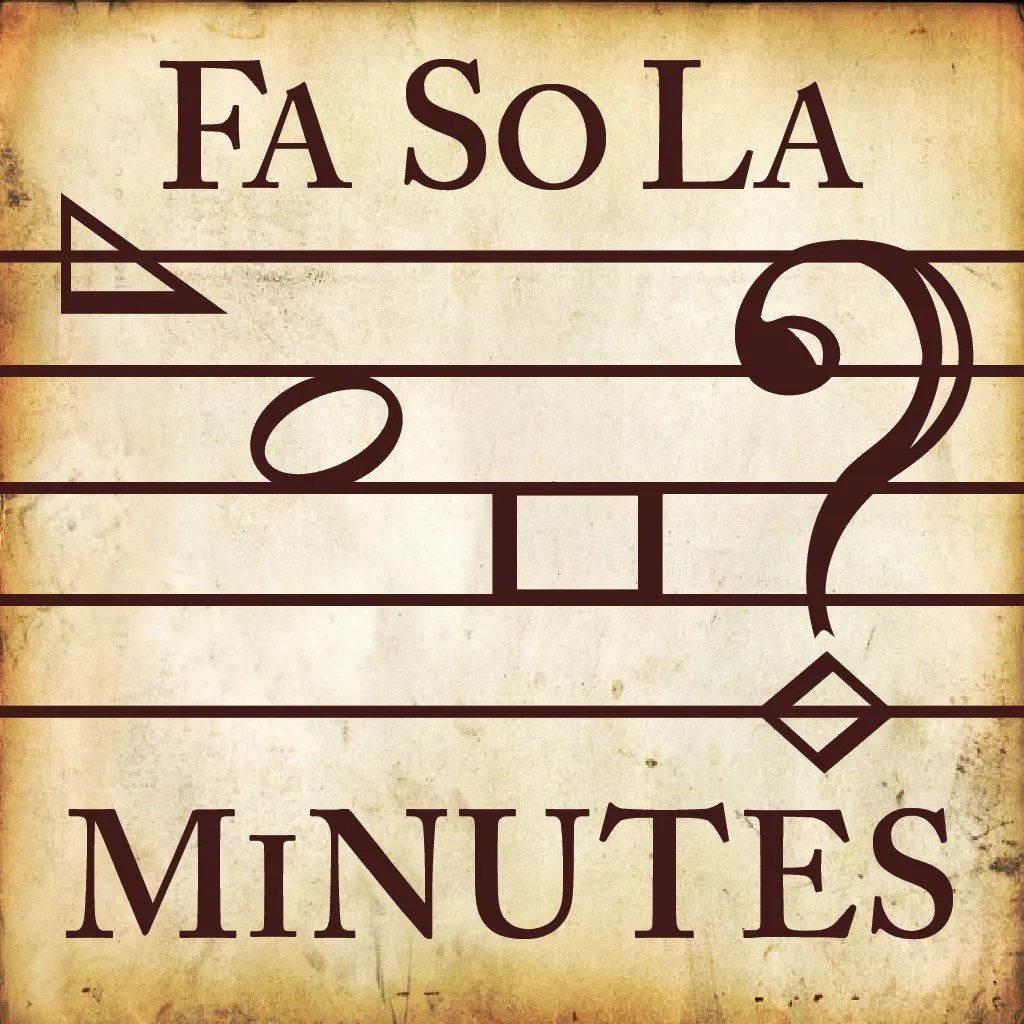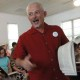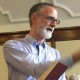# Entropy Unpacked: The Entropy Number from “FaSoLa Minutes”

### IntroductionSince its initial release in 2013, the “FaSoLa Minutes” iOS app has become an important component of how many singers learn and engage with Sacred Harp songs, singers, and singings. The app includes data from the Minutes of Sacred Harp Singings since 1995. A review of the app appeared in vol. 3, no. 2 of this newsletter. Among many useful features described in that review, the app can be used to answer statistical questions such as:

• Which songs in The Sacred Harp: 1991 Edition have been led the most often?
• How has the popularity of each song varied over time?
• How many times has each leader led since 1995, and how many different songs?
• What is each leader’s entropy number?

…and this is the point in many discussions of the “FaSoLa Minutes” app where the puzzled expressions appear, because the entropy measure is unfamiliar to most Sacred Harp singers. You may have some additional questions:

• Why is the entropy measure included with the other statistics in the app?
• How is the entropy number calculated?
• How does my choice of songs change my entropy number?
• Does my entropy measure matter—and should I work to change it?

We will answer these questions with both nontechnical explanations and formulas for readers who are so inclined. In the following sections, we describe what the entropy number is intended to measure and how to interpret it. Then we explain the formulas used to define entropy with simple examples that illustrate how song choice affects the entropy measurement. We conclude with thoughts on how song leaders can use the entropy number, with tables that illustrate how entropy is affected based on a leader’s choice of songs.Information poster for the “Fa So La Minutes” app, designed by Lauren Bock, at the 2013 National Convention. Photograph by Robert Chambless.

### How to Interpret the Entropy Number

The “Help” page in the “FaSoLa Minutes” app describes entropy as “a measure from 1 to 0 of a singer’s unpredictability when leading.” An entropy number near 0 indicates that a leader is very predictable, while a number near 1 indicates high unpredictability.

Note that the entropy number does not indicate whether someone is a good leader. For example, one of the qualities of a good leader is the ability to choose an appropriate song considering such factors as the time of day and the energy of the class. A leader who is unpredictable to the point of being completely random is unlikely to do this well. Also, the statistics in the app are based on song choices in the minutes, and therefore don’t reflect other recognized qualities of a good leader, such as familiarity with the song one chooses to lead, selection of appropriate verses and tempo, and good communication of the leader’s intentions to the class. There are excellent leaders with relatively high entropy, and there are other excellent leaders with relatively low entropy.

### History and Mathematical Formula for Entropy

The formula for entropy was developed by Claude Shannon in the 1940s. Shannon was a mathematician at Bell Labs, the research and development branch of the Bell Telephone Company. His ideas of information theory became part of the foundation of computer science. Shannon defined the smallest unit of information as a “bit,” which can only have one of two values:$\boldsymbol{0}$ or$\boldsymbol{1}$. He described how any message can be encoded as a sequence of bits, and developed the entropy formula as a way to measure the amount of information in a message. Shannon entropy “$\boldsymbol{H}$” is defined by this formula:$\boldsymbol{H= -\sum p_i log_2(p_i)}$

where:$\boldsymbol{p_i}$ is the probability of a particular term appearing in the message, out of all the possible terms—in our case, the probability of leading a particular song (song$\boldsymbol{i}$) from The Sacred Harp.$\boldsymbol{log_2}$ is a logarithm to base 2. (Logarithms are the reverse of exponentiation. We say that the logarithm of 8 to base 2, written$\boldsymbol{log_2(8)}$, is equal to 3 because$\boldsymbol{{2}^{3} = 8}$.) Shannon
used base 2 in this formula because the “bit” has two possible values.

The symbol$\boldsymbol{\sum}$ (the Greek letter Sigma) is mathematical shorthand for a sum of a number of terms, where in this case each term is$\boldsymbol{p_i log_2(p_i)}$ with the index value “$\boldsymbol{i}$.” So the entropy formula could also be written as:$\boldsymbol{H= -[p_1 log_2(p_1) + p_2 log_2(p_2) + p_3 log_2(p_3) + ... ]}$

including a separate term for each song, but that would take much longer to write out.

For the entropy calculation in the app, we make the assumption that the frequency each song has been lead in the past is likely to predict future behavior. So, for example, if you’ve led 100 times in the minutes, and 20 of those were “Lenox” (p. 40), then
the frequency with which you led “Lenox” was 0.2, or 20 percent, and we would use 20 percent as the probability$\boldsymbol{p_i}$ for “Lenox.”

For each leader, the probabilities of leading each song in the book need to be added together to calculate that leader’s entropy. If you’ve never led a particular song, it neither adds to nor subtracts from your entropy.

The maximum possible entropy comes from choosing any of the 554 songs in The Sacred Harp: 1991 Edition, with equal probability. In other words, the class has no way of predicting which song you are about to call, so the leader with maximum entropy is completely unpredictable. In math terms, all the$\boldsymbol{p_i}$’s would be the same, 1/554. The Shannon entropy of the completely unpredictable leader would be:$\boldsymbol{H = -554 (1/554) log_2(1/554) = -log_2(1/554) = 9.114}$

In the “FaSoLa Minutes” app, someone who is completely predictable (i.e. always leads the same song) has an entropy of 0, and the completely unpredictable leader has an entropy of 1.0. This is because entropy reported in the app has been “normalized” by dividing by$\boldsymbol{-log_2(1/554) = 9.114}$. (Normalization is frequently used in statistics, because it makes it easier to identify where a statistical value falls between the minimum (0 or 0%) and maximum (1 or 100%) possible values.) A reported (“relative”) entropy of 0.600 is actually a raw entropy of 5.47, divided by 9.114, the maximum possible entropy.

“FaSoLa Minutes” app normalized entropy$\boldsymbol{= -\sum p_i log_2(p_i)/-log_2(1/554)}$

### Example: How Song Choice Affects Entropy Number

Say a leader, Catherine, has led 2 different songs 3 times: “Lenox” twice, and “New
Britain” (p. 45t) once.

Then in the calculation of the entropy formula, “Lenox” has a probability of 2/3, and “New Britain” has a probability of 1/3, so the entropy number is 0.1008. (If you look through the leaders listed in the app, you will find many leaders who have led two different songs three times and therefore have this entropy number.)

Now Catherine is going to lead for a fourth time, and is choosing a song. How will her entropy number change based on her choice?

Option 1: If she leads “Lenox” again, its probability rises to 3/4, and “New Britain’s” drops to 1/4. This makes her song choice more predictable than before, so her new entropy number goes down to 0.0890.

Option 2: If she leads “New Britain” again, then its probability rises to 2/4, and “Lenox’s” probability falls to 2/4. Her entropy rises to 0.1097, because her song choice is less predictable than before, even though she has still only led 2 songs.

Option 3: She picks a new song to lead—for the formula it doesn’t matter which of the other 552 songs in The Sacred Harp she chooses. Then “Lenox’s” probability drops to
2/4, “New Britain’s” drops to 1/4, and the new song has probability 1/4. As a result, her entropy rises to 0.1646, because her song choice is much less predictable than before.

In general, leading a song that was previously led could either increase or decrease the entropy number, depending on how it changes the probabilities of the songs already led, but leading a new song always results in the largest change in entropy, and it always increases the entropy number.

(Homework for the interested reader: Using some algebra and the properties of logarithms, the formula for “FaSoLa Minutes” app entropy shown above simplifies to$\boldsymbol{E = \sum p_i log(p_i)/log(1/554)}$, which uses common logarithms instead of the logarithm to base 2. Common logarithms are a common function on scientific calculators, so this makes it possible to work out simple examples such as the ones above with a calculator. Give it a try!)

### How Song Leaders Use Entropy

So what does entropy mean for song leaders? One way to think about it is in terms of the number of songs a singer seems to be leading. If a leader has led 20 different songs one time each, her entropy would be 0.48. If she has led 50 different songs, her entropy would be 0.63. See Table 1 below for some sample figures. If a leader led the number of songs on the left, one time each, that leader would have the entropy number on the right.

Table 2 shows the equivalent number of songs, led one time each, which correspond to a given entropy number in the app. This gives a sense of the minimum number of songs/times an individual would have to lead to arrive at the corresponding entropy.

Note that because almost all leaders have repeated songs, and most leaders lead some songs more often than other songs, most leaders who have led 20 different songs will have an entropy lower than the maximum 0.48; this difference between the actual entropy for a leader and the highest possible entropy in these tables indicates indirectly how often a leader has chosen to repeat a previously-led song, as opposed to trying a new one.

### Some ObservationsThis graph of Table 2 shows the non-linear relationship between the entropy number and required minimum number of songs led (with each song being led one time).

You’ll notice from the tables that the result isn’t “linear”: as the entropy number increases, it takes a lot more songs to get the same amount of change in entropy.

### Conclusion

We hope that this article improves understanding of the entropy statistic in the “FaSoLa Minutes” app, and appreciation for all the components that make one a good leader. Attaining a high entropy number is not a useful end in itself, but the entropy measure provides information that can help us think about how we choose songs to lead.

The iOS application “FaSoLa Minutes” was developed by Mark Godfrey, and designed by Lauren Bock. It is available for \$4.99 from the iPhone App Store, with proceeds going to the Sacred Harp Musical Heritage Association.

### Tables

Table 1
Minimum Number of Songs Entropy
4 0.219
8 0.329
16 0.439
32 0.549
64 0.658
128 0.768
256 0.878
Table 2
Entropy Minimum Number of Songs
0.30 7
0.35 9
0.40 13
0.45 17
0.50 24
0.55 32
0.60 44
0.65 61
0.70 83
0.75 114
0.80 157
0.85 215
0.90 295
0.95 404
1.00 554David Brodeur is a highly entropic leader from Atlanta, Georgia. He has been singing Sacred Harp since 2011.David Smead lives in Decatur, Georgia. He works with mathematical models and has taught mathematics to college students. David has been singing Sacred Harp since 2013.
This entry was posted in Number, Measure, Weight. Bookmark the permalink.

### 7 Responses to Entropy Unpacked: The Entropy Number from “FaSoLa Minutes”

1.Micah Walter says:

I’m wondering whether there could be a different way to measure probability. If I’ve led only one or two songs, the app’s entropy measure assumes that those are the only songs I might lead in the future. But in reality, if I’ve only led once, that gives no information about my future probability—I might never lead them again. It’s only when I’ve begun to lead more often that it becomes clear that the probabilities of those songs are actually higher.

So if I’ve led 5 songs 5 times, the proportion of songs led to times led implies that my probability of leading those songs again is actually lower. The problem is quantifying this intuition.

•David Brodeur says:

You pose good questions about an intuitive understanding of entropy in a series of song choices. Rather than thinking of our ability to predict someone’s next lesson (since they may choose any song in the book if it hasn’t been led that day), think of how much information about their choices is contained in the record of prior lessons. There is no entropy in one lesson of one song (entropy equals zero), since the frequency of that lesson is one. Likewise, there is no entropy in leading the same song over and over, no matter how many times (again the frequency of that song will be one). A more illuminating case is the one you cite: What If I lead five songs, one time each? This is the maximally entropic way to lead five times, with an entropy of 0.255. Any repeats among the songs, for a total of five lessons, would lead to a lower entropy measure, and convey the intuition that the leader is a bit more predictable, i.e., less “random.”

Note again that there is a maximal attainable entropy for any particular number of lessons, i.e., by leading a new song every time. Those maxima are detailed in the Tables. Your sense is correct that we know more about a leader after they have led fifty times than we do after they have led five times: as the number of lessons increases, the range of possible outcomes increases. Try comparing the entropy of a leader to their maximum attainable entropy for that total number of lessons. This gives a better relative sense of their predictability.

•Micah Walter says:

This is good advice! Somehow I missed the tables on my first reading. I’m wondering how valuable it would be to “normalize” entropy by listing the quotient of actual entropy/possible entropy for number songs. Do you think this would be a good intuitive measurement?

•David Brodeur says:

I think that quotient is a good way to make fair comparisons. I don’t know that that means it will be added to the app.

2.Gerry Hoffman says:

At the risk of appearing to be a smarty pants, I’ll point out that Claude Shannon’s formula for entropy of information is essentially identical to an older formula for what is called “entropy of mixing” in chemistry—except for the base of the logarithm.

•David Brodeur says:

It wasn’t lost on Shannon and his colleagues in the 1940s that their formulation of information entropy resembled entropy in statistical mechanics, as worked out by Gibbs, Boltzmann, and others in the nineteenth century. This is probably why they adopted the term.

Anecdotally, Shannon was told that using the term entropy was ideal, because nobody really understood entropy anyway, and he could say whatever he wanted without fear of contradiction.

3.Sam says:

I would love it if something like this were available for desktop computer users at some point.